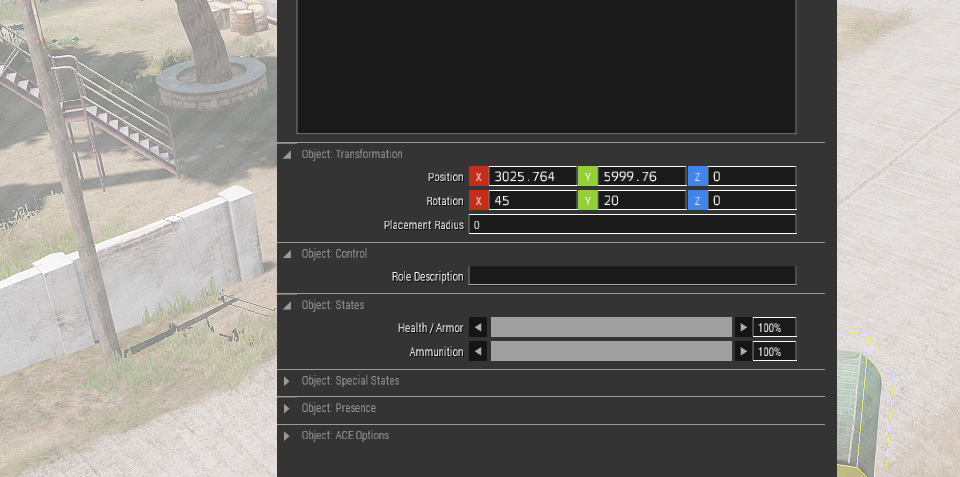# Getting Eden Rotations from Objects

The Eden editor controls the orientation of objects using the rotation attribute, which, if you are developing scripts in Eden, can be accessed using get3DENAttribute and set3DENAttributes.

If you have an object within a running mission—or you somehow have one in Eden that isn't an entity—you can't just read the rotations directly to put them back into Eden. Existing functions to read rotations from objects aren't much help either; they typically use a different order for the rotations, or a different sign for the angles.

The following function implements the conversion, taking in to account that Eden uses an ZYX rotation order, and that it has—when compared with traditional rotation matrices—each of the rotation angles negated:

```fhcb_getEdenAngles =
{
private _direction = vectorDir _this;
private _up = vectorUp _this;
private _aside = _direction vectorCrossProduct _up;

private ["_xRot", "_yRot", "_zRot"];

if (abs (_up select 0) < 0.999999) then {
_yRot = -asin (_up select 0);

private _signCosY = if (cos _yRot < 0) then { -1 } else { 1 };

_xRot = (_up select 1 * _signCosY) atan2 (_up select 2 * _signCosY);
_zRot = (_direction select 0 * _signCosY) atan2 (_aside select 0 * _signCosY);
} else {
_zRot = 0;

if (_up select 0 < 0) then {
_yRot = 90;
_xRot = (_aside select 1) atan2 (_aside select 2);
} else {
_yRot = -90;
_xRot = (-(_aside select 1)) atan2 (-(_aside select 2));
};
};

[_xRot, _yRot, _zRot]
};
```

To use it, simply call it with the object as the parameter:

```private _angles = someObject call fhcb_getEdenAngles;

_angles params ["_xRot", "_yRot", "_zRot"];
```